# Ch8_slides.ppt

29. May 2023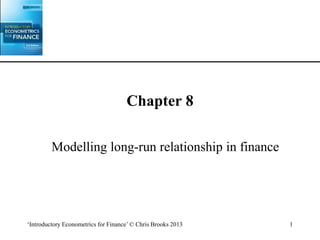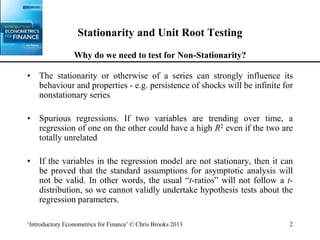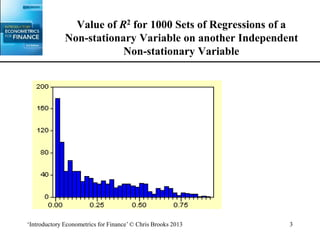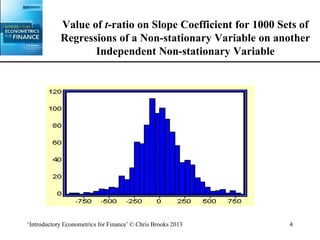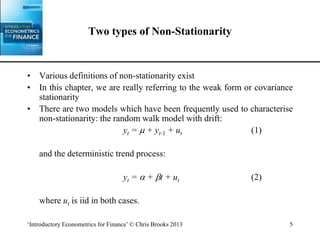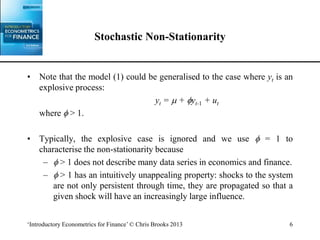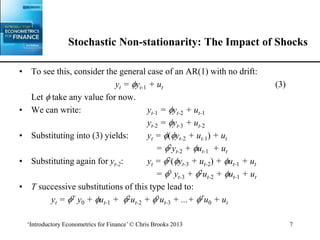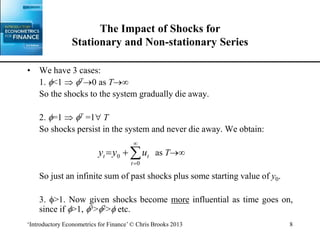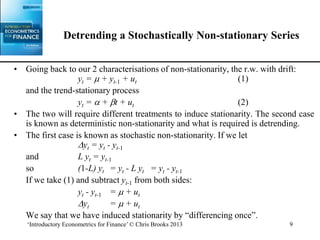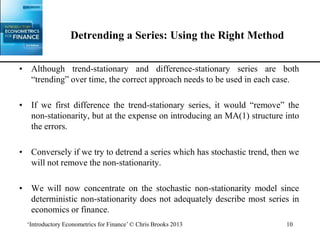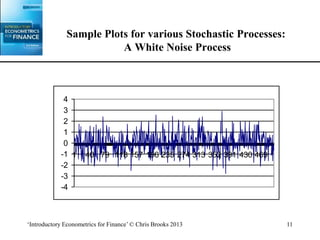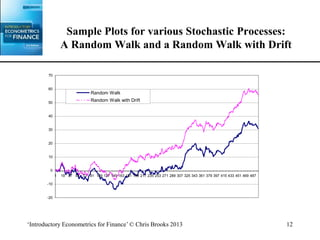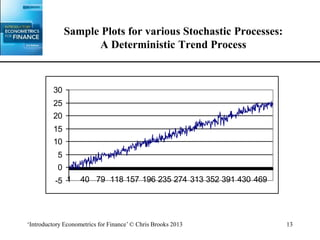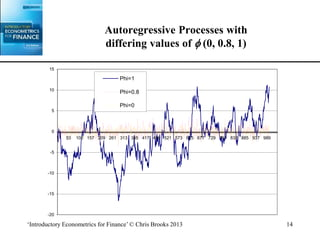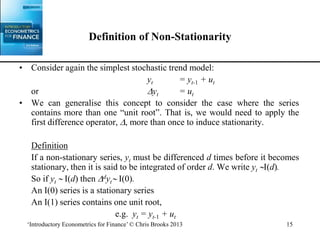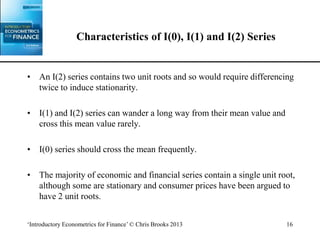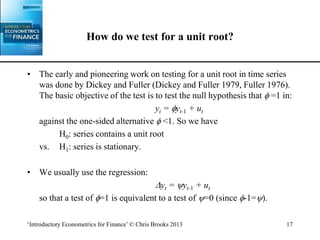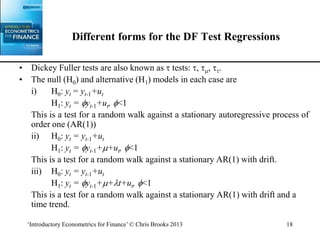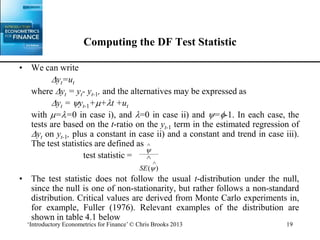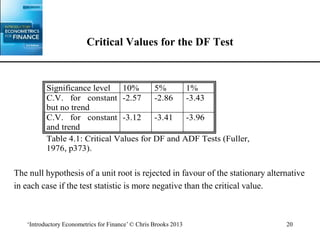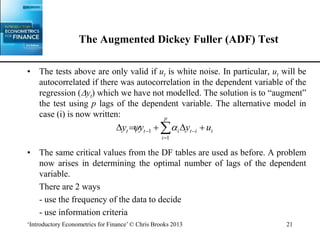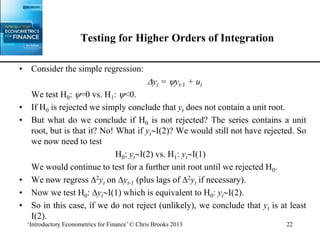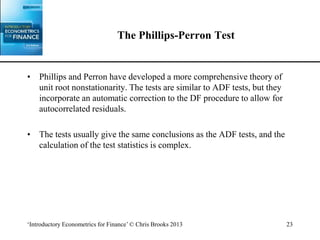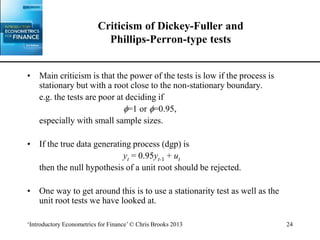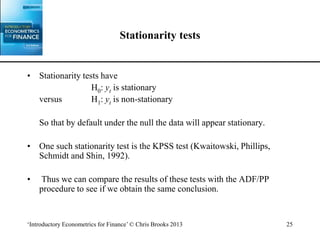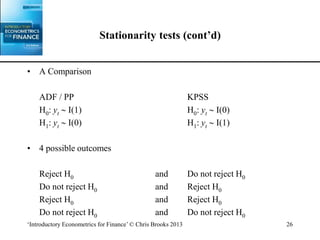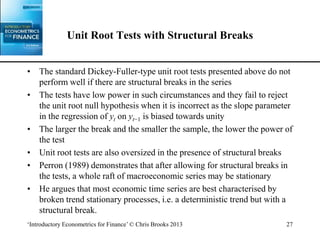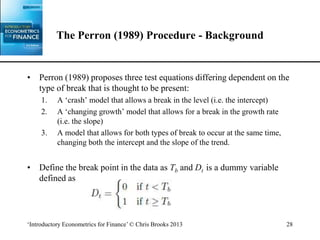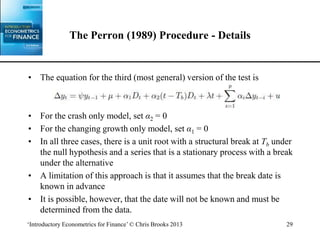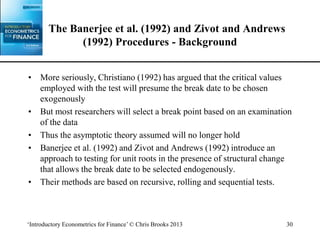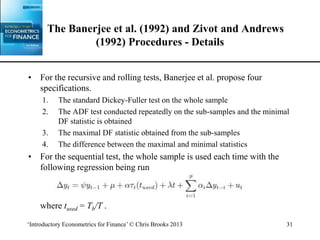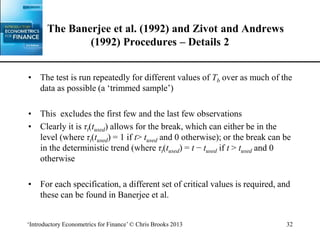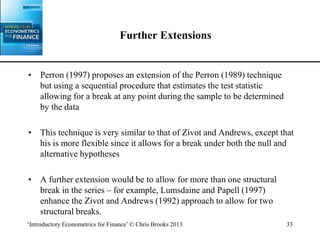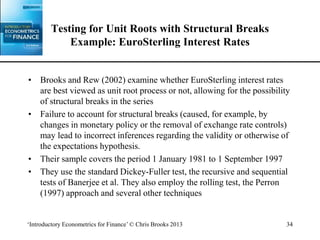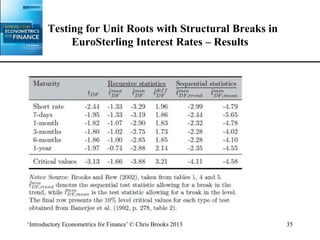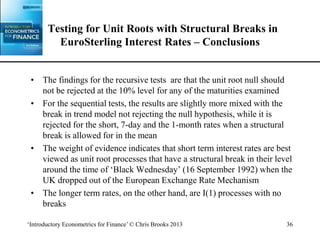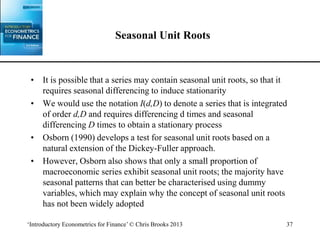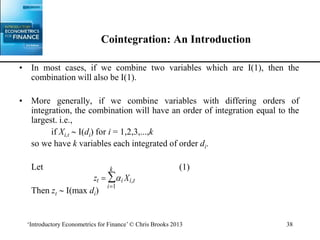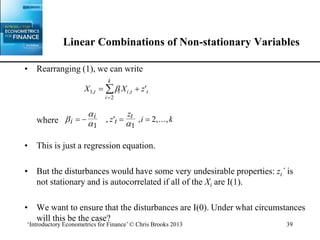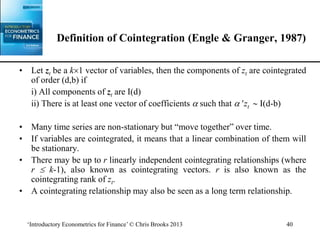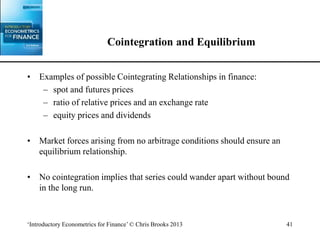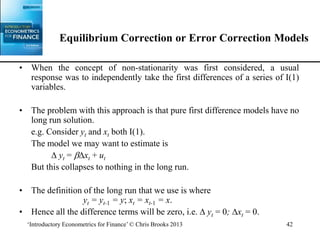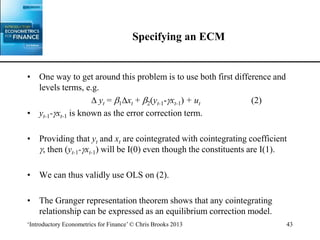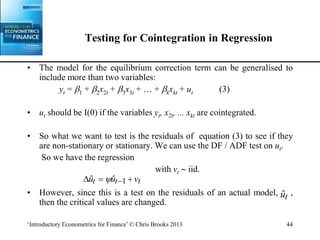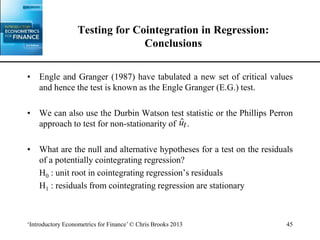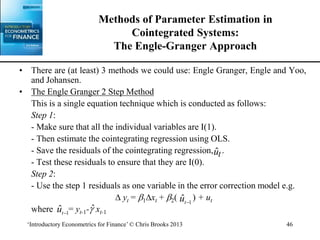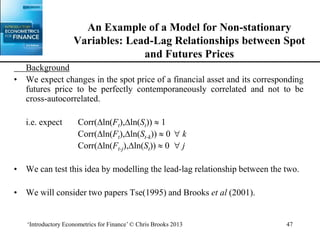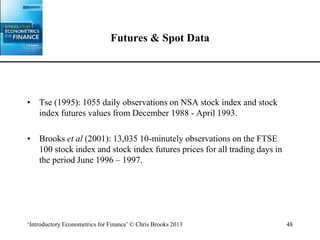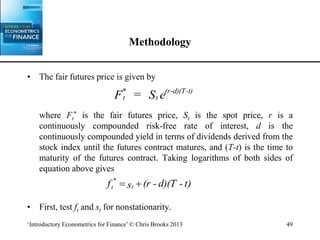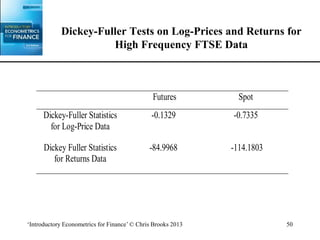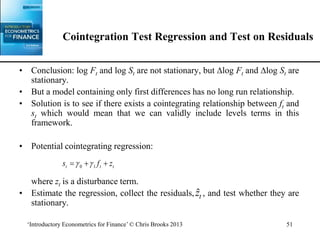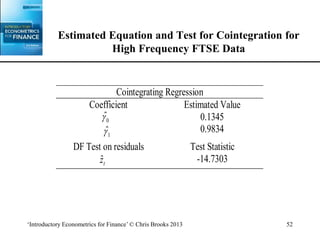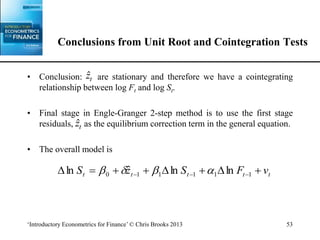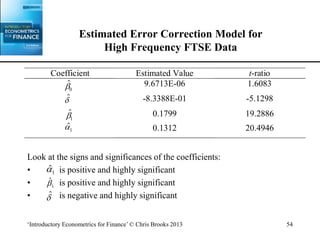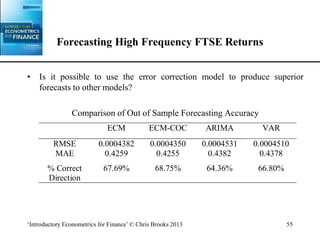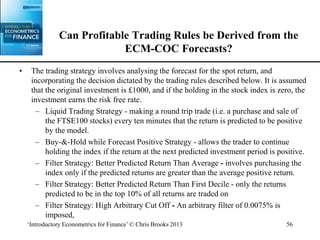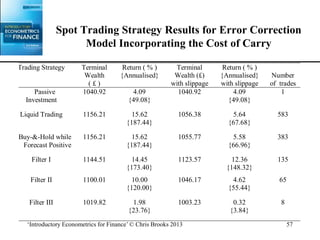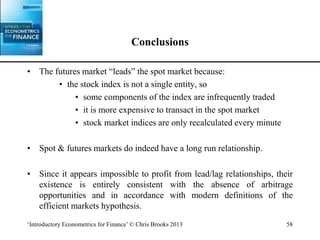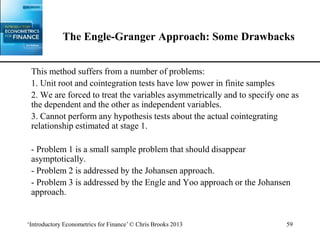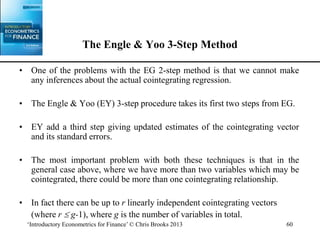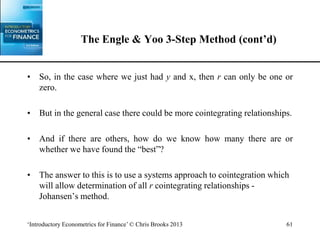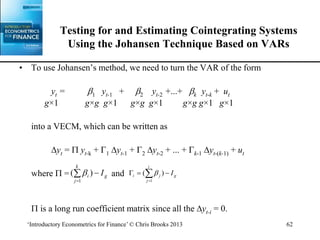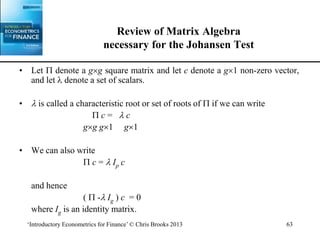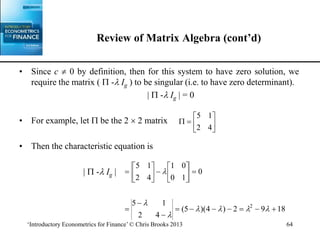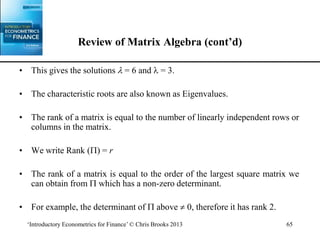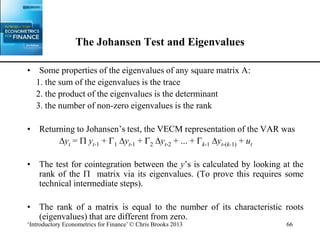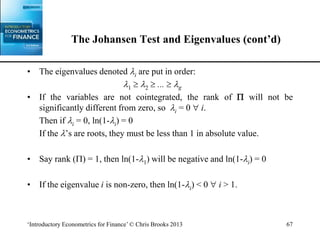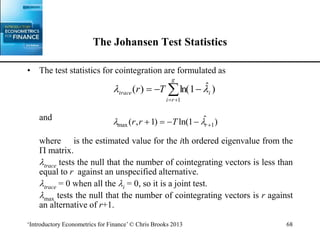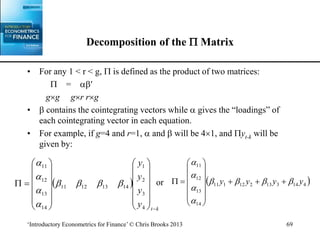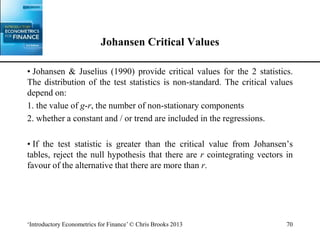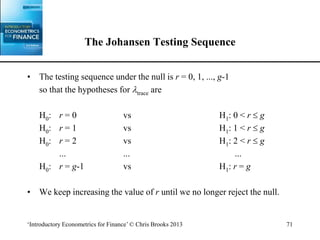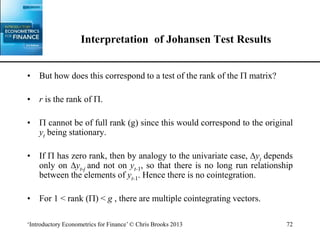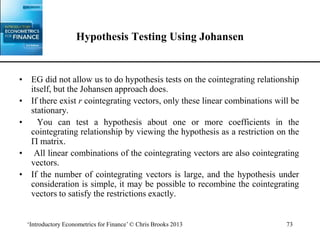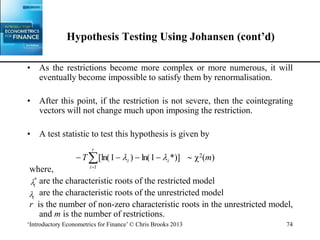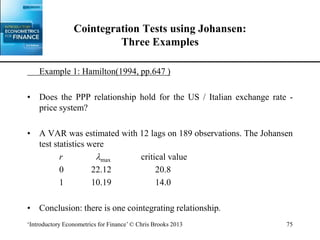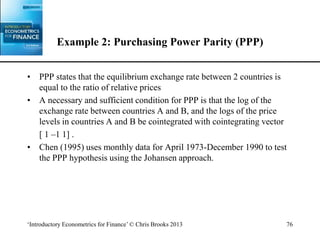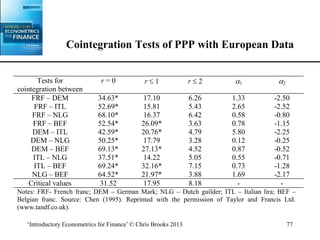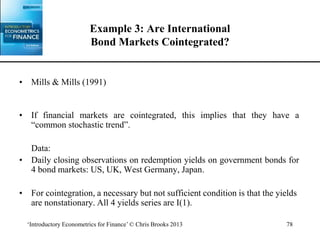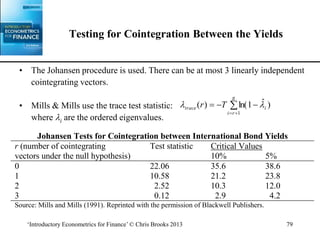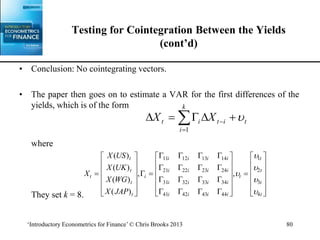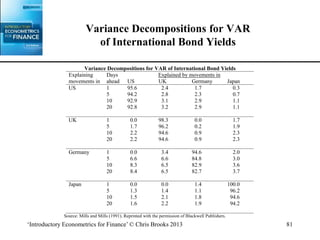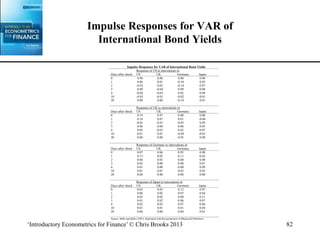1 von 82

### Ch8_slides.ppt

• 1. ‘Introductory Econometrics for Finance’ © Chris Brooks 2013 1 Chapter 8 Modelling long-run relationship in finance
• 2. ‘Introductory Econometrics for Finance’ © Chris Brooks 2013 2 Stationarity and Unit Root Testing Why do we need to test for Non-Stationarity? • The stationarity or otherwise of a series can strongly influence its behaviour and properties - e.g. persistence of shocks will be infinite for nonstationary series • Spurious regressions. If two variables are trending over time, a regression of one on the other could have a high R2 even if the two are totally unrelated • If the variables in the regression model are not stationary, then it can be proved that the standard assumptions for asymptotic analysis will not be valid. In other words, the usual “t-ratios” will not follow a t- distribution, so we cannot validly undertake hypothesis tests about the regression parameters.
• 3. ‘Introductory Econometrics for Finance’ © Chris Brooks 2013 3 Value of R2 for 1000 Sets of Regressions of a Non-stationary Variable on another Independent Non-stationary Variable
• 4. ‘Introductory Econometrics for Finance’ © Chris Brooks 2013 4 Value of t-ratio on Slope Coefficient for 1000 Sets of Regressions of a Non-stationary Variable on another Independent Non-stationary Variable
• 5. ‘Introductory Econometrics for Finance’ © Chris Brooks 2013 5 Two types of Non-Stationarity • Various definitions of non-stationarity exist • In this chapter, we are really referring to the weak form or covariance stationarity • There are two models which have been frequently used to characterise non-stationarity: the random walk model with drift: yt =  + yt-1 + ut (1) and the deterministic trend process: yt =  + t + ut (2) where ut is iid in both cases.
• 6. ‘Introductory Econometrics for Finance’ © Chris Brooks 2013 6 Stochastic Non-Stationarity • Note that the model (1) could be generalised to the case where yt is an explosive process: yt =  + yt-1 + ut where  > 1. • Typically, the explosive case is ignored and we use  = 1 to characterise the non-stationarity because –  > 1 does not describe many data series in economics and finance. –  > 1 has an intuitively unappealing property: shocks to the system are not only persistent through time, they are propagated so that a given shock will have an increasingly large influence.
• 7. ‘Introductory Econometrics for Finance’ © Chris Brooks 2013 7 Stochastic Non-stationarity: The Impact of Shocks • To see this, consider the general case of an AR(1) with no drift: yt = yt-1 + ut (3) Let  take any value for now. • We can write: yt-1 = yt-2 + ut-1 yt-2 = yt-3 + ut-2 • Substituting into (3) yields: yt = (yt-2 + ut-1) + ut = 2yt-2 + ut-1 + ut • Substituting again for yt-2: yt = 2(yt-3 + ut-2) + ut-1 + ut = 3 yt-3 + 2ut-2 + ut-1 + ut • T successive substitutions of this type lead to: yt = T y0 + ut-1 + 2ut-2 + 3ut-3 + ...+ Tu0 + ut
• 8. ‘Introductory Econometrics for Finance’ © Chris Brooks 2013 8 The Impact of Shocks for Stationary and Non-stationary Series • We have 3 cases: 1. <1  T0 as T So the shocks to the system gradually die away. 2. =1  T =1 T So shocks persist in the system and never die away. We obtain: as T So just an infinite sum of past shocks plus some starting value of y0. 3. >1. Now given shocks become more influential as time goes on, since if >1, 3>2> etc.      0 0 t t t u y y
• 9. ‘Introductory Econometrics for Finance’ © Chris Brooks 2013 9 Detrending a Stochastically Non-stationary Series • Going back to our 2 characterisations of non-stationarity, the r.w. with drift: yt =  + yt-1 + ut (1) and the trend-stationary process yt =  + t + ut (2) • The two will require different treatments to induce stationarity. The second case is known as deterministic non-stationarity and what is required is detrending. • The first case is known as stochastic non-stationarity. If we let yt = yt - yt-1 and L yt = yt-1 so (1-L) yt = yt - L yt = yt - yt-1 If we take (1) and subtract yt-1 from both sides: yt - yt-1 =  + ut yt =  + ut We say that we have induced stationarity by “differencing once”.
• 10. ‘Introductory Econometrics for Finance’ © Chris Brooks 2013 10 Detrending a Series: Using the Right Method • Although trend-stationary and difference-stationary series are both “trending” over time, the correct approach needs to be used in each case. • If we first difference the trend-stationary series, it would “remove” the non-stationarity, but at the expense on introducing an MA(1) structure into the errors. • Conversely if we try to detrend a series which has stochastic trend, then we will not remove the non-stationarity. • We will now concentrate on the stochastic non-stationarity model since deterministic non-stationarity does not adequately describe most series in economics or finance.
• 11. ‘Introductory Econometrics for Finance’ © Chris Brooks 2013 11 Sample Plots for various Stochastic Processes: A White Noise Process -4 -3 -2 -1 0 1 2 3 4 1 40 79 118 157 196 235 274 313 352 391 430 469
• 12. ‘Introductory Econometrics for Finance’ © Chris Brooks 2013 12 Sample Plots for various Stochastic Processes: A Random Walk and a Random Walk with Drift -20 -10 0 10 20 30 40 50 60 70 1 19 37 55 73 91 109 127 145 163 181 199 217 235 253 271 289 307 325 343 361 379 397 415 433 451 469 487 Random Walk Random Walk with Drift
• 13. ‘Introductory Econometrics for Finance’ © Chris Brooks 2013 13 Sample Plots for various Stochastic Processes: A Deterministic Trend Process -5 0 5 10 15 20 25 30 1 40 79 118 157 196 235 274 313 352 391 430 469
• 14. ‘Introductory Econometrics for Finance’ © Chris Brooks 2013 14 Autoregressive Processes with differing values of  (0, 0.8, 1) -20 -15 -10 -5 0 5 10 15 1 53 105 157 209 261 313 365 417 469 521 573 625 677 729 781 833 885 937 989 Phi=1 Phi=0.8 Phi=0
• 15. ‘Introductory Econometrics for Finance’ © Chris Brooks 2013 15 Definition of Non-Stationarity • Consider again the simplest stochastic trend model: yt = yt-1 + ut or yt = ut • We can generalise this concept to consider the case where the series contains more than one “unit root”. That is, we would need to apply the first difference operator, , more than once to induce stationarity. Definition If a non-stationary series, yt must be differenced d times before it becomes stationary, then it is said to be integrated of order d. We write yt I(d). So if yt  I(d) then dyt I(0). An I(0) series is a stationary series An I(1) series contains one unit root, e.g. yt = yt-1 + ut
• 16. ‘Introductory Econometrics for Finance’ © Chris Brooks 2013 16 Characteristics of I(0), I(1) and I(2) Series • An I(2) series contains two unit roots and so would require differencing twice to induce stationarity. • I(1) and I(2) series can wander a long way from their mean value and cross this mean value rarely. • I(0) series should cross the mean frequently. • The majority of economic and financial series contain a single unit root, although some are stationary and consumer prices have been argued to have 2 unit roots.
• 17. ‘Introductory Econometrics for Finance’ © Chris Brooks 2013 17 How do we test for a unit root? • The early and pioneering work on testing for a unit root in time series was done by Dickey and Fuller (Dickey and Fuller 1979, Fuller 1976). The basic objective of the test is to test the null hypothesis that  =1 in: yt = yt-1 + ut against the one-sided alternative  <1. So we have H0: series contains a unit root vs. H1: series is stationary. • We usually use the regression: yt = yt-1 + ut so that a test of =1 is equivalent to a test of =0 (since -1=).
• 18. ‘Introductory Econometrics for Finance’ © Chris Brooks 2013 18 Different forms for the DF Test Regressions • Dickey Fuller tests are also known as  tests: , , . • The null (H0) and alternative (H1) models in each case are i) H0: yt = yt-1+ut H1: yt = yt-1+ut, <1 This is a test for a random walk against a stationary autoregressive process of order one (AR(1)) ii) H0: yt = yt-1+ut H1: yt = yt-1++ut, <1 This is a test for a random walk against a stationary AR(1) with drift. iii) H0: yt = yt-1+ut H1: yt = yt-1++t+ut, <1 This is a test for a random walk against a stationary AR(1) with drift and a time trend.
• 19. ‘Introductory Econometrics for Finance’ © Chris Brooks 2013 19 Computing the DF Test Statistic • We can write yt=ut where yt = yt- yt-1, and the alternatives may be expressed as yt = yt-1++t +ut with ==0 in case i), and =0 in case ii) and =-1. In each case, the tests are based on the t-ratio on the yt-1 term in the estimated regression of yt on yt-1, plus a constant in case ii) and a constant and trend in case iii). The test statistics are defined as test statistic = • The test statistic does not follow the usual t-distribution under the null, since the null is one of non-stationarity, but rather follows a non-standard distribution. Critical values are derived from Monte Carlo experiments in, for example, Fuller (1976). Relevant examples of the distribution are shown in table 4.1 below      SE( )
• 20. ‘Introductory Econometrics for Finance’ © Chris Brooks 2013 20 Critical Values for the DF Test The null hypothesis of a unit root is rejected in favour of the stationary alternative in each case if the test statistic is more negative than the critical value. Significance level 10% 5% 1% C.V. for constant but no trend -2.57 -2.86 -3.43 C.V. for constant and trend -3.12 -3.41 -3.96 Table 4.1: Critical Values for DF and ADF Tests (Fuller, 1976, p373).
• 21. ‘Introductory Econometrics for Finance’ © Chris Brooks 2013 21 The Augmented Dickey Fuller (ADF) Test • The tests above are only valid if ut is white noise. In particular, ut will be autocorrelated if there was autocorrelation in the dependent variable of the regression (yt) which we have not modelled. The solution is to “augment” the test using p lags of the dependent variable. The alternative model in case (i) is now written: • The same critical values from the DF tables are used as before. A problem now arises in determining the optimal number of lags of the dependent variable. There are 2 ways - use the frequency of the data to decide - use information criteria          p i t i t i t t u y y y 1 1  
• 22. ‘Introductory Econometrics for Finance’ © Chris Brooks 2013 22 Testing for Higher Orders of Integration • Consider the simple regression: yt = yt-1 + ut We test H0: =0 vs. H1: <0. • If H0 is rejected we simply conclude that yt does not contain a unit root. • But what do we conclude if H0 is not rejected? The series contains a unit root, but is that it? No! What if ytI(2)? We would still not have rejected. So we now need to test H0: ytI(2) vs. H1: ytI(1) We would continue to test for a further unit root until we rejected H0. • We now regress 2yt on yt-1 (plus lags of 2yt if necessary). • Now we test H0: ytI(1) which is equivalent to H0: ytI(2). • So in this case, if we do not reject (unlikely), we conclude that yt is at least I(2).
• 23. ‘Introductory Econometrics for Finance’ © Chris Brooks 2013 23 The Phillips-Perron Test • Phillips and Perron have developed a more comprehensive theory of unit root nonstationarity. The tests are similar to ADF tests, but they incorporate an automatic correction to the DF procedure to allow for autocorrelated residuals. • The tests usually give the same conclusions as the ADF tests, and the calculation of the test statistics is complex.
• 24. ‘Introductory Econometrics for Finance’ © Chris Brooks 2013 24 Criticism of Dickey-Fuller and Phillips-Perron-type tests • Main criticism is that the power of the tests is low if the process is stationary but with a root close to the non-stationary boundary. e.g. the tests are poor at deciding if =1 or =0.95, especially with small sample sizes. • If the true data generating process (dgp) is yt = 0.95yt-1 + ut then the null hypothesis of a unit root should be rejected. • One way to get around this is to use a stationarity test as well as the unit root tests we have looked at.
• 25. ‘Introductory Econometrics for Finance’ © Chris Brooks 2013 25 Stationarity tests • Stationarity tests have H0: yt is stationary versus H1: yt is non-stationary So that by default under the null the data will appear stationary. • One such stationarity test is the KPSS test (Kwaitowski, Phillips, Schmidt and Shin, 1992). • Thus we can compare the results of these tests with the ADF/PP procedure to see if we obtain the same conclusion.
• 26. ‘Introductory Econometrics for Finance’ © Chris Brooks 2013 26 Stationarity tests (cont’d) • A Comparison ADF / PP KPSS H0: yt  I(1) H0: yt  I(0) H1: yt  I(0) H1: yt  I(1) • 4 possible outcomes Reject H0 and Do not reject H0 Do not reject H0 and Reject H0 Reject H0 and Reject H0 Do not reject H0 and Do not reject H0
• 27. ‘Introductory Econometrics for Finance’ © Chris Brooks 2013 27 Unit Root Tests with Structural Breaks • The standard Dickey-Fuller-type unit root tests presented above do not perform well if there are structural breaks in the series • The tests have low power in such circumstances and they fail to reject the unit root null hypothesis when it is incorrect as the slope parameter in the regression of yt on yt−1 is biased towards unity • The larger the break and the smaller the sample, the lower the power of the test • Unit root tests are also oversized in the presence of structural breaks • Perron (1989) demonstrates that after allowing for structural breaks in the tests, a whole raft of macroeconomic series may be stationary • He argues that most economic time series are best characterised by broken trend stationary processes, i.e. a deterministic trend but with a structural break.
• 28. ‘Introductory Econometrics for Finance’ © Chris Brooks 2013 28 The Perron (1989) Procedure - Background • Perron (1989) proposes three test equations differing dependent on the type of break that is thought to be present: 1. A ‘crash’ model that allows a break in the level (i.e. the intercept) 2. A ‘changing growth’ model that allows for a break in the growth rate (i.e. the slope) 3. A model that allows for both types of break to occur at the same time, changing both the intercept and the slope of the trend. • Define the break point in the data as Tb and Dt is a dummy variable defined as
• 29. ‘Introductory Econometrics for Finance’ © Chris Brooks 2013 29 The Perron (1989) Procedure - Details • The equation for the third (most general) version of the test is • For the crash only model, set α2 = 0 • For the changing growth only model, set α1 = 0 • In all three cases, there is a unit root with a structural break at Tb under the null hypothesis and a series that is a stationary process with a break under the alternative • A limitation of this approach is that it assumes that the break date is known in advance • It is possible, however, that the date will not be known and must be determined from the data.
• 30. ‘Introductory Econometrics for Finance’ © Chris Brooks 2013 30 The Banerjee et al. (1992) and Zivot and Andrews (1992) Procedures - Background • More seriously, Christiano (1992) has argued that the critical values employed with the test will presume the break date to be chosen exogenously • But most researchers will select a break point based on an examination of the data • Thus the asymptotic theory assumed will no longer hold • Banerjee et al. (1992) and Zivot and Andrews (1992) introduce an approach to testing for unit roots in the presence of structural change that allows the break date to be selected endogenously. • Their methods are based on recursive, rolling and sequential tests.
• 31. ‘Introductory Econometrics for Finance’ © Chris Brooks 2013 31 The Banerjee et al. (1992) and Zivot and Andrews (1992) Procedures - Details • For the recursive and rolling tests, Banerjee et al. propose four specifications. 1. The standard Dickey-Fuller test on the whole sample 2. The ADF test conducted repeatedly on the sub-samples and the minimal DF statistic is obtained 3. The maximal DF statistic obtained from the sub-samples 4. The difference between the maximal and minimal statistics • For the sequential test, the whole sample is used each time with the following regression being run where tused = Tb/T .
• 32. ‘Introductory Econometrics for Finance’ © Chris Brooks 2013 32 The Banerjee et al. (1992) and Zivot and Andrews (1992) Procedures – Details 2 • The test is run repeatedly for different values of Tb over as much of the data as possible (a ‘trimmed sample’) • This excludes the first few and the last few observations • Clearly it is τt(tused) allows for the break, which can either be in the level (where τt(tused) = 1 if t> tused and 0 otherwise); or the break can be in the deterministic trend (where τt(tused) = t − tused if t > tused and 0 otherwise • For each specification, a different set of critical values is required, and these can be found in Banerjee et al.
• 33. ‘Introductory Econometrics for Finance’ © Chris Brooks 2013 33 Further Extensions • Perron (1997) proposes an extension of the Perron (1989) technique but using a sequential procedure that estimates the test statistic allowing for a break at any point during the sample to be determined by the data • This technique is very similar to that of Zivot and Andrews, except that his is more flexible since it allows for a break under both the null and alternative hypotheses • A further extension would be to allow for more than one structural break in the series – for example, Lumsdaine and Papell (1997) enhance the Zivot and Andrews (1992) approach to allow for two structural breaks.
• 34. ‘Introductory Econometrics for Finance’ © Chris Brooks 2013 34 Testing for Unit Roots with Structural Breaks Example: EuroSterling Interest Rates • Brooks and Rew (2002) examine whether EuroSterling interest rates are best viewed as unit root process or not, allowing for the possibility of structural breaks in the series • Failure to account for structural breaks (caused, for example, by changes in monetary policy or the removal of exchange rate controls) may lead to incorrect inferences regarding the validity or otherwise of the expectations hypothesis. • Their sample covers the period 1 January 1981 to 1 September 1997 • They use the standard Dickey-Fuller test, the recursive and sequential tests of Banerjee et al. They also employ the rolling test, the Perron (1997) approach and several other techniques
• 35. ‘Introductory Econometrics for Finance’ © Chris Brooks 2013 35 Testing for Unit Roots with Structural Breaks in EuroSterling Interest Rates – Results
• 36. ‘Introductory Econometrics for Finance’ © Chris Brooks 2013 36 Testing for Unit Roots with Structural Breaks in EuroSterling Interest Rates – Conclusions • The findings for the recursive tests are that the unit root null should not be rejected at the 10% level for any of the maturities examined • For the sequential tests, the results are slightly more mixed with the break in trend model not rejecting the null hypothesis, while it is rejected for the short, 7-day and the 1-month rates when a structural break is allowed for in the mean • The weight of evidence indicates that short term interest rates are best viewed as unit root processes that have a structural break in their level around the time of ‘Black Wednesday’ (16 September 1992) when the UK dropped out of the European Exchange Rate Mechanism • The longer term rates, on the other hand, are I(1) processes with no breaks
• 37. ‘Introductory Econometrics for Finance’ © Chris Brooks 2013 37 Seasonal Unit Roots • It is possible that a series may contain seasonal unit roots, so that it requires seasonal differencing to induce stationarity • We would use the notation I(d,D) to denote a series that is integrated of order d,D and requires differencing d times and seasonal differencing D times to obtain a stationary process • Osborn (1990) develops a test for seasonal unit roots based on a natural extension of the Dickey-Fuller approach. • However, Osborn also shows that only a small proportion of macroeconomic series exhibit seasonal unit roots; the majority have seasonal patterns that can better be characterised using dummy variables, which may explain why the concept of seasonal unit roots has not been widely adopted
• 38. ‘Introductory Econometrics for Finance’ © Chris Brooks 2013 38 Cointegration: An Introduction • In most cases, if we combine two variables which are I(1), then the combination will also be I(1). • More generally, if we combine variables with differing orders of integration, the combination will have an order of integration equal to the largest. i.e., if Xi,t  I(di) for i = 1,2,3,...,k so we have k variables each integrated of order di. Let (1) Then zt  I(max di) z X t i i t i k    , 1
• 39. ‘Introductory Econometrics for Finance’ © Chris Brooks 2013 39 Linear Combinations of Non-stationary Variables • Rearranging (1), we can write where • This is just a regression equation. • But the disturbances would have some very undesirable properties: zt´ is not stationary and is autocorrelated if all of the Xi are I(1). • We want to ensure that the disturbances are I(0). Under what circumstances will this be the case?     i i t t z z i k     1 1 2 , ' , ,..., X X z t i i t t i k 1 2 , , '    
• 40. ‘Introductory Econometrics for Finance’ © Chris Brooks 2013 40 Definition of Cointegration (Engle & Granger, 1987) • Let zt be a k1 vector of variables, then the components of zt are cointegrated of order (d,b) if i) All components of zt are I(d) ii) There is at least one vector of coefficients  such that  zt  I(d-b) • Many time series are non-stationary but “move together” over time. • If variables are cointegrated, it means that a linear combination of them will be stationary. • There may be up to r linearly independent cointegrating relationships (where r  k-1), also known as cointegrating vectors. r is also known as the cointegrating rank of zt. • A cointegrating relationship may also be seen as a long term relationship.
• 41. ‘Introductory Econometrics for Finance’ © Chris Brooks 2013 41 Cointegration and Equilibrium • Examples of possible Cointegrating Relationships in finance: – spot and futures prices – ratio of relative prices and an exchange rate – equity prices and dividends • Market forces arising from no arbitrage conditions should ensure an equilibrium relationship. • No cointegration implies that series could wander apart without bound in the long run.
• 42. ‘Introductory Econometrics for Finance’ © Chris Brooks 2013 42 Equilibrium Correction or Error Correction Models • When the concept of non-stationarity was first considered, a usual response was to independently take the first differences of a series of I(1) variables. • The problem with this approach is that pure first difference models have no long run solution. e.g. Consider yt and xt both I(1). The model we may want to estimate is  yt = xt + ut But this collapses to nothing in the long run. • The definition of the long run that we use is where yt = yt-1 = y; xt = xt-1 = x. • Hence all the difference terms will be zero, i.e.  yt = 0; xt = 0.
• 43. ‘Introductory Econometrics for Finance’ © Chris Brooks 2013 43 Specifying an ECM • One way to get around this problem is to use both first difference and levels terms, e.g.  yt = 1xt + 2(yt-1-xt-1) + ut (2) • yt-1-xt-1 is known as the error correction term. • Providing that yt and xt are cointegrated with cointegrating coefficient , then (yt-1-xt-1) will be I(0) even though the constituents are I(1). • We can thus validly use OLS on (2). • The Granger representation theorem shows that any cointegrating relationship can be expressed as an equilibrium correction model.
• 44. ‘Introductory Econometrics for Finance’ © Chris Brooks 2013 44 Testing for Cointegration in Regression • The model for the equilibrium correction term can be generalised to include more than two variables: yt = 1 + 2x2t + 3x3t + … + kxkt + ut (3) • ut should be I(0) if the variables yt, x2t, ... xkt are cointegrated. • So what we want to test is the residuals of equation (3) to see if they are non-stationary or stationary. We can use the DF / ADF test on ut. So we have the regression with vt  iid. • However, since this is a test on the residuals of an actual model, , then the critical values are changed.   u u v t t t     1  ut
• 45. ‘Introductory Econometrics for Finance’ © Chris Brooks 2013 45 Testing for Cointegration in Regression: Conclusions • Engle and Granger (1987) have tabulated a new set of critical values and hence the test is known as the Engle Granger (E.G.) test. • We can also use the Durbin Watson test statistic or the Phillips Perron approach to test for non-stationarity of . • What are the null and alternative hypotheses for a test on the residuals of a potentially cointegrating regression? H0 : unit root in cointegrating regression’s residuals H1 : residuals from cointegrating regression are stationary  ut
• 46. ‘Introductory Econometrics for Finance’ © Chris Brooks 2013 46 Methods of Parameter Estimation in Cointegrated Systems: The Engle-Granger Approach • There are (at least) 3 methods we could use: Engle Granger, Engle and Yoo, and Johansen. • The Engle Granger 2 Step Method This is a single equation technique which is conducted as follows: Step 1: - Make sure that all the individual variables are I(1). - Then estimate the cointegrating regression using OLS. - Save the residuals of the cointegrating regression, . - Test these residuals to ensure that they are I(0). Step 2: - Use the step 1 residuals as one variable in the error correction model e.g.  yt = 1xt + 2( ) + ut where = yt-1- xt-1 1 ˆ  t u 1 ˆ  t u  ut ˆ
• 47. ‘Introductory Econometrics for Finance’ © Chris Brooks 2013 47 An Example of a Model for Non-stationary Variables: Lead-Lag Relationships between Spot and Futures Prices Background • We expect changes in the spot price of a financial asset and its corresponding futures price to be perfectly contemporaneously correlated and not to be cross-autocorrelated. i.e. expect Corr(ln(Ft),ln(St))  1 Corr(ln(Ft),ln(St-k))  0  k Corr(ln(Ft-j),ln(St))  0  j • We can test this idea by modelling the lead-lag relationship between the two. • We will consider two papers Tse(1995) and Brooks et al (2001).
• 48. ‘Introductory Econometrics for Finance’ © Chris Brooks 2013 48 Futures & Spot Data • Tse (1995): 1055 daily observations on NSA stock index and stock index futures values from December 1988 - April 1993. • Brooks et al (2001): 13,035 10-minutely observations on the FTSE 100 stock index and stock index futures prices for all trading days in the period June 1996 – 1997.
• 49. ‘Introductory Econometrics for Finance’ © Chris Brooks 2013 49 Methodology • The fair futures price is given by where Ft * is the fair futures price, St is the spot price, r is a continuously compounded risk-free rate of interest, d is the continuously compounded yield in terms of dividends derived from the stock index until the futures contract matures, and (T-t) is the time to maturity of the futures contract. Taking logarithms of both sides of equation above gives • First, test ft and st for nonstationarity. t * t (r-d)(T-t) F = S e t) - d)(T - (r s f t t   *
• 50. ‘Introductory Econometrics for Finance’ © Chris Brooks 2013 50 Dickey-Fuller Tests on Log-Prices and Returns for High Frequency FTSE Data Futures Spot Dickey-Fuller Statistics for Log-Price Data -0.1329 -0.7335 Dickey Fuller Statistics for Returns Data -84.9968 -114.1803
• 51. ‘Introductory Econometrics for Finance’ © Chris Brooks 2013 51 Cointegration Test Regression and Test on Residuals • Conclusion: log Ft and log St are not stationary, but log Ft and log St are stationary. • But a model containing only first differences has no long run relationship. • Solution is to see if there exists a cointegrating relationship between ft and st which would mean that we can validly include levels terms in this framework. • Potential cointegrating regression: where zt is a disturbance term. • Estimate the regression, collect the residuals, , and test whether they are stationary.  zt t t t z f s    1 0  
• 52. ‘Introductory Econometrics for Finance’ © Chris Brooks 2013 52 Estimated Equation and Test for Cointegration for High Frequency FTSE Data Cointegrating Regression Coefficient  0  1 Estimated Value 0.1345 0.9834 DF Test on residuals t ẑ Test Statistic -14.7303
• 53. ‘Introductory Econometrics for Finance’ © Chris Brooks 2013 53 Conclusions from Unit Root and Cointegration Tests • Conclusion: are stationary and therefore we have a cointegrating relationship between log Ft and log St. • Final stage in Engle-Granger 2-step method is to use the first stage residuals, as the equilibrium correction term in the general equation. • The overall model is  zt  zt t t t t t v F S z S            1 1 1 1 1 0 ln ln ˆ ln    
• 54. ‘Introductory Econometrics for Finance’ © Chris Brooks 2013 54 Estimated Error Correction Model for High Frequency FTSE Data Look at the signs and significances of the coefficients: • is positive and highly significant • is positive and highly significant • is negative and highly significant Coefficient Estimated Value t-ratio  0 9.6713E-06 1.6083   -8.3388E-01 -5.1298  1 0.1799 19.2886  1 0.1312 20.4946 1 ̂ 1 ̂  
• 55. ‘Introductory Econometrics for Finance’ © Chris Brooks 2013 55 Forecasting High Frequency FTSE Returns • Is it possible to use the error correction model to produce superior forecasts to other models? Comparison of Out of Sample Forecasting Accuracy ECM ECM-COC ARIMA VAR RMSE 0.0004382 0.0004350 0.0004531 0.0004510 MAE 0.4259 0.4255 0.4382 0.4378 % Correct Direction 67.69% 68.75% 64.36% 66.80%
• 56. ‘Introductory Econometrics for Finance’ © Chris Brooks 2013 56 Can Profitable Trading Rules be Derived from the ECM-COC Forecasts? • The trading strategy involves analysing the forecast for the spot return, and incorporating the decision dictated by the trading rules described below. It is assumed that the original investment is £1000, and if the holding in the stock index is zero, the investment earns the risk free rate. – Liquid Trading Strategy - making a round trip trade (i.e. a purchase and sale of the FTSE100 stocks) every ten minutes that the return is predicted to be positive by the model. – Buy-&-Hold while Forecast Positive Strategy - allows the trader to continue holding the index if the return at the next predicted investment period is positive. – Filter Strategy: Better Predicted Return Than Average - involves purchasing the index only if the predicted returns are greater than the average positive return. – Filter Strategy: Better Predicted Return Than First Decile - only the returns predicted to be in the top 10% of all returns are traded on – Filter Strategy: High Arbitrary Cut Off - An arbitrary filter of 0.0075% is imposed,
• 57. ‘Introductory Econometrics for Finance’ © Chris Brooks 2013 57 Trading Strategy Terminal Wealth ( £ ) Return ( % ) {Annualised} Terminal Wealth (£) with slippage Return ( % ) {Annualised} with slippage Number of trades Passive Investment 1040.92 4.09 {49.08} 1040.92 4.09 {49.08} 1 Liquid Trading 1156.21 15.62 {187.44} 1056.38 5.64 {67.68} 583 Buy-&-Hold while Forecast Positive 1156.21 15.62 {187.44} 1055.77 5.58 {66.96} 383 Filter I 1144.51 14.45 {173.40} 1123.57 12.36 {148.32} 135 Filter II 1100.01 10.00 {120.00} 1046.17 4.62 {55.44} 65 Filter III 1019.82 1.98 {23.76} 1003.23 0.32 {3.84} 8 Spot Trading Strategy Results for Error Correction Model Incorporating the Cost of Carry
• 58. ‘Introductory Econometrics for Finance’ © Chris Brooks 2013 58 Conclusions • The futures market “leads” the spot market because: • the stock index is not a single entity, so • some components of the index are infrequently traded • it is more expensive to transact in the spot market • stock market indices are only recalculated every minute • Spot & futures markets do indeed have a long run relationship. • Since it appears impossible to profit from lead/lag relationships, their existence is entirely consistent with the absence of arbitrage opportunities and in accordance with modern definitions of the efficient markets hypothesis.
• 59. ‘Introductory Econometrics for Finance’ © Chris Brooks 2013 59 The Engle-Granger Approach: Some Drawbacks This method suffers from a number of problems: 1. Unit root and cointegration tests have low power in finite samples 2. We are forced to treat the variables asymmetrically and to specify one as the dependent and the other as independent variables. 3. Cannot perform any hypothesis tests about the actual cointegrating relationship estimated at stage 1. - Problem 1 is a small sample problem that should disappear asymptotically. - Problem 2 is addressed by the Johansen approach. - Problem 3 is addressed by the Engle and Yoo approach or the Johansen approach.
• 60. ‘Introductory Econometrics for Finance’ © Chris Brooks 2013 60 • One of the problems with the EG 2-step method is that we cannot make any inferences about the actual cointegrating regression. • The Engle & Yoo (EY) 3-step procedure takes its first two steps from EG. • EY add a third step giving updated estimates of the cointegrating vector and its standard errors. • The most important problem with both these techniques is that in the general case above, where we have more than two variables which may be cointegrated, there could be more than one cointegrating relationship. • In fact there can be up to r linearly independent cointegrating vectors (where r  g-1), where g is the number of variables in total. The Engle & Yoo 3-Step Method
• 61. ‘Introductory Econometrics for Finance’ © Chris Brooks 2013 61 • So, in the case where we just had y and x, then r can only be one or zero. • But in the general case there could be more cointegrating relationships. • And if there are others, how do we know how many there are or whether we have found the “best”? • The answer to this is to use a systems approach to cointegration which will allow determination of all r cointegrating relationships - Johansen’s method. The Engle & Yoo 3-Step Method (cont’d)
• 62. ‘Introductory Econometrics for Finance’ © Chris Brooks 2013 62 • To use Johansen’s method, we need to turn the VAR of the form yt = 1 yt-1 + 2 yt-2 +...+ k yt-k + ut g×1 g×g g×1 g×g g×1 g×g g×1 g×1 into a VECM, which can be written as yt =  yt-k + 1 yt-1 + 2 yt-2 + ... + k-1 yt-(k-1) + ut where  = and  is a long run coefficient matrix since all the yt-i = 0. Testing for and Estimating Cointegrating Systems Using the Johansen Technique Based on VARs    k j g i I 1 ) (       i j g j i I 1 ) ( 
• 63. ‘Introductory Econometrics for Finance’ © Chris Brooks 2013 63 • Let  denote a gg square matrix and let c denote a g1 non-zero vector, and let  denote a set of scalars. •  is called a characteristic root or set of roots of  if we can write  c =  c gg g1 g1 • We can also write  c =  Ip c and hence (  - Ig ) c = 0 where Ig is an identity matrix. Review of Matrix Algebra necessary for the Johansen Test
• 64. ‘Introductory Econometrics for Finance’ © Chris Brooks 2013 64 • Since c  0 by definition, then for this system to have zero solution, we require the matrix (  - Ig ) to be singular (i.e. to have zero determinant).   - Ig  = 0 • For example, let  be the 2  2 matrix • Then the characteristic equation is   - Ig  Review of Matrix Algebra (cont’d)         5 1 2 4                          5 1 2 4 1 0 0 1 0 5 1 2 4 5 4 2 9 18 2        ( )( )
• 65. ‘Introductory Econometrics for Finance’ © Chris Brooks 2013 65 • This gives the solutions  = 6 and  = 3. • The characteristic roots are also known as Eigenvalues. • The rank of a matrix is equal to the number of linearly independent rows or columns in the matrix. • We write Rank () = r • The rank of a matrix is equal to the order of the largest square matrix we can obtain from  which has a non-zero determinant. • For example, the determinant of  above  0, therefore it has rank 2. Review of Matrix Algebra (cont’d)
• 66. ‘Introductory Econometrics for Finance’ © Chris Brooks 2013 66 • Some properties of the eigenvalues of any square matrix A: 1. the sum of the eigenvalues is the trace 2. the product of the eigenvalues is the determinant 3. the number of non-zero eigenvalues is the rank • Returning to Johansen’s test, the VECM representation of the VAR was yt =  yt-1 + 1 yt-1 + 2 yt-2 + ... + k-1 yt-(k-1) + ut • The test for cointegration between the y’s is calculated by looking at the rank of the  matrix via its eigenvalues. (To prove this requires some technical intermediate steps). • The rank of a matrix is equal to the number of its characteristic roots (eigenvalues) that are different from zero. The Johansen Test and Eigenvalues
• 67. ‘Introductory Econometrics for Finance’ © Chris Brooks 2013 67 • The eigenvalues denoted i are put in order: 1  2  ...  g • If the variables are not cointegrated, the rank of  will not be significantly different from zero, so i = 0  i. Then if i = 0, ln(1-i) = 0 If the ’s are roots, they must be less than 1 in absolute value. • Say rank () = 1, then ln(1-1) will be negative and ln(1-i) = 0 • If the eigenvalue i is non-zero, then ln(1-i) < 0  i > 1. The Johansen Test and Eigenvalues (cont’d)
• 68. ‘Introductory Econometrics for Finance’ © Chris Brooks 2013 68 • The test statistics for cointegration are formulated as and where is the estimated value for the ith ordered eigenvalue from the  matrix. trace tests the null that the number of cointegrating vectors is less than equal to r against an unspecified alternative. trace = 0 when all the i = 0, so it is a joint test. max tests the null that the number of cointegrating vectors is r against an alternative of r+1. The Johansen Test Statistics   max ( , ) ln(  ) r r T r      1 1 1       g r i i trace T r 1 ) ˆ 1 ln( ) (  
• 69. ‘Introductory Econometrics for Finance’ © Chris Brooks 2013 69 Decomposition of the  Matrix • For any 1 < r < g,  is defined as the product of two matrices:  =  gg gr rg •  contains the cointegrating vectors while  gives the “loadings” of each cointegrating vector in each equation. • For example, if g=4 and r=1,  and  will be 41, and yt-k will be given by: or   k t y y y y                                4 3 2 1 14 13 12 11 14 13 12 11           4 14 3 13 2 12 1 11 14 13 12 11 y y y y                           
• 70. ‘Introductory Econometrics for Finance’ © Chris Brooks 2013 70 • Johansen & Juselius (1990) provide critical values for the 2 statistics. The distribution of the test statistics is non-standard. The critical values depend on: 1. the value of g-r, the number of non-stationary components 2. whether a constant and / or trend are included in the regressions. • If the test statistic is greater than the critical value from Johansen’s tables, reject the null hypothesis that there are r cointegrating vectors in favour of the alternative that there are more than r. Johansen Critical Values
• 71. ‘Introductory Econometrics for Finance’ © Chris Brooks 2013 71 • The testing sequence under the null is r = 0, 1, ..., g-1 so that the hypotheses for trace are H0: r = 0 vs H1: 0 < r  g H0: r = 1 vs H1: 1 < r  g H0: r = 2 vs H1: 2 < r  g ... ... ... H0: r = g-1 vs H1: r = g • We keep increasing the value of r until we no longer reject the null. The Johansen Testing Sequence
• 72. ‘Introductory Econometrics for Finance’ © Chris Brooks 2013 72 • But how does this correspond to a test of the rank of the  matrix? • r is the rank of . •  cannot be of full rank (g) since this would correspond to the original yt being stationary. • If  has zero rank, then by analogy to the univariate case, yt depends only on yt-j and not on yt-1, so that there is no long run relationship between the elements of yt-1. Hence there is no cointegration. • For 1 < rank () < g , there are multiple cointegrating vectors. Interpretation of Johansen Test Results
• 73. ‘Introductory Econometrics for Finance’ © Chris Brooks 2013 73 Hypothesis Testing Using Johansen • EG did not allow us to do hypothesis tests on the cointegrating relationship itself, but the Johansen approach does. • If there exist r cointegrating vectors, only these linear combinations will be stationary. • You can test a hypothesis about one or more coefficients in the cointegrating relationship by viewing the hypothesis as a restriction on the  matrix. • All linear combinations of the cointegrating vectors are also cointegrating vectors. • If the number of cointegrating vectors is large, and the hypothesis under consideration is simple, it may be possible to recombine the cointegrating vectors to satisfy the restrictions exactly.
• 74. ‘Introductory Econometrics for Finance’ © Chris Brooks 2013 74 Hypothesis Testing Using Johansen (cont’d) • As the restrictions become more complex or more numerous, it will eventually become impossible to satisfy them by renormalisation. • After this point, if the restriction is not severe, then the cointegrating vectors will not change much upon imposing the restriction. • A test statistic to test this hypothesis is given by  2(m) where, are the characteristic roots of the restricted model are the characteristic roots of the unrestricted model r is the number of non-zero characteristic roots in the unrestricted model, and m is the number of restrictions. i * i       r i i i T 1 *)] 1 ln( ) 1 [ln(  
• 75. ‘Introductory Econometrics for Finance’ © Chris Brooks 2013 75 Cointegration Tests using Johansen: Three Examples Example 1: Hamilton(1994, pp.647 ) • Does the PPP relationship hold for the US / Italian exchange rate - price system? • A VAR was estimated with 12 lags on 189 observations. The Johansen test statistics were r max critical value 0 22.12 20.8 1 10.19 14.0 • Conclusion: there is one cointegrating relationship.
• 76. ‘Introductory Econometrics for Finance’ © Chris Brooks 2013 76 Example 2: Purchasing Power Parity (PPP) • PPP states that the equilibrium exchange rate between 2 countries is equal to the ratio of relative prices • A necessary and sufficient condition for PPP is that the log of the exchange rate between countries A and B, and the logs of the price levels in countries A and B be cointegrated with cointegrating vector [ 1 –1 1] . • Chen (1995) uses monthly data for April 1973-December 1990 to test the PPP hypothesis using the Johansen approach.
• 77. ‘Introductory Econometrics for Finance’ © Chris Brooks 2013 77 Cointegration Tests of PPP with European Data Tests for cointegration between r = 0 r  1 r  2 1 2 FRF – DEM 34.63* 17.10 6.26 1.33 -2.50 FRF – ITL 52.69* 15.81 5.43 2.65 -2.52 FRF – NLG 68.10* 16.37 6.42 0.58 -0.80 FRF – BEF 52.54* 26.09* 3.63 0.78 -1.15 DEM – ITL 42.59* 20.76* 4.79 5.80 -2.25 DEM – NLG 50.25* 17.79 3.28 0.12 -0.25 DEM – BEF 69.13* 27.13* 4.52 0.87 -0.52 ITL – NLG 37.51* 14.22 5.05 0.55 -0.71 ITL – BEF 69.24* 32.16* 7.15 0.73 -1.28 NLG – BEF 64.52* 21.97* 3.88 1.69 -2.17 Critical values 31.52 17.95 8.18 - - Notes: FRF- French franc; DEM – German Mark; NLG – Dutch guilder; ITL – Italian lira; BEF – Belgian franc. Source: Chen (1995). Reprinted with the permission of Taylor and Francis Ltd. (www.tandf.co.uk).
• 78. ‘Introductory Econometrics for Finance’ © Chris Brooks 2013 78 Example 3: Are International Bond Markets Cointegrated? • Mills & Mills (1991) • If financial markets are cointegrated, this implies that they have a “common stochastic trend”. Data: • Daily closing observations on redemption yields on government bonds for 4 bond markets: US, UK, West Germany, Japan. • For cointegration, a necessary but not sufficient condition is that the yields are nonstationary. All 4 yields series are I(1).
• 79. ‘Introductory Econometrics for Finance’ © Chris Brooks 2013 79 Testing for Cointegration Between the Yields • The Johansen procedure is used. There can be at most 3 linearly independent cointegrating vectors. • Mills & Mills use the trace test statistic: where i are the ordered eigenvalues.       g r i i trace T r 1 ) ˆ 1 ln( ) (   Johansen Tests for Cointegration between International Bond Yields Test statistic Critical Values r (number of cointegrating vectors under the null hypothesis) 10% 5% 0 22.06 35.6 38.6 1 10.58 21.2 23.8 2 2.52 10.3 12.0 3 0.12 2.9 4.2 Source: Mills and Mills (1991). Reprinted with the permission of Blackwell Publishers.
• 80. ‘Introductory Econometrics for Finance’ © Chris Brooks 2013 80 Testing for Cointegration Between the Yields (cont’d) • Conclusion: No cointegrating vectors. • The paper then goes on to estimate a VAR for the first differences of the yields, which is of the form where They set k = 8. X X US X UK X WG X JAP t t t t t i i i i i i i i i i i i i i i i i t t t t t                                        ( ) ( ) ( ) ( ) , ,                  11 12 13 14 21 22 23 24 31 32 33 34 41 42 43 44 1 2 3 4              k i t i t i t X X 1 
• 81. ‘Introductory Econometrics for Finance’ © Chris Brooks 2013 81 Variance Decompositions for VAR of International Bond Yields Variance Decompositions for VAR of International Bond Yields Explained by movements in Explaining movements in Days ahead US UK Germany Japan US 1 95.6 2.4 1.7 0.3 5 94.2 2.8 2.3 0.7 10 92.9 3.1 2.9 1.1 20 92.8 3.2 2.9 1.1 UK 1 0.0 98.3 0.0 1.7 5 1.7 96.2 0.2 1.9 10 2.2 94.6 0.9 2.3 20 2.2 94.6 0.9 2.3 Germany 1 0.0 3.4 94.6 2.0 5 6.6 6.6 84.8 3.0 10 8.3 6.5 82.9 3.6 20 8.4 6.5 82.7 3.7 Japan 1 0.0 0.0 1.4 100.0 5 1.3 1.4 1.1 96.2 10 1.5 2.1 1.8 94.6 20 1.6 2.2 1.9 94.2 Source: Mills and Mills (1991). Reprinted with the permission of Blackwell Publishers.
• 82. ‘Introductory Econometrics for Finance’ © Chris Brooks 2013 82 Impulse Responses for VAR of International Bond Yields Impulse Responses for VAR of International Bond Yields Response of US to innovations in Days after shock US UK Germany Japan 0 0.98 0.00 0.00 0.00 1 0.06 0.01 -0.10 0.05 2 -0.02 0.02 -0.14 0.07 3 0.09 -0.04 0.09 0.08 4 -0.02 -0.03 0.02 0.09 10 -0.03 -0.01 -0.02 -0.01 20 0.00 0.00 -0.10 -0.01 Response of UK to innovations in Days after shock US UK Germany Japan 0 0.19 0.97 0.00 0.00 1 0.16 0.07 0.01 -0.06 2 -0.01 -0.01 -0.05 0.09 3 0.06 0.04 0.06 0.05 4 0.05 -0.01 0.02 0.07 10 0.01 0.01 -0.04 -0.01 20 0.00 0.00 -0.01 0.00 Response of Germany to innovations in Days after shock US UK Germany Japan 0 0.07 0.06 0.95 0.00 1 0.13 0.05 0.11 0.02 2 0.04 0.03 0.00 0.00 3 0.02 0.00 0.00 0.01 4 0.01 0.00 0.00 0.09 10 0.01 0.01 -0.01 0.02 20 0.00 0.00 0.00 0.00 Response of Japan to innovations in Days after shock US UK Germany Japan 0 0.03 0.05 0.12 0.97 1 0.06 0.02 0.07 0.04 2 0.02 0.02 0.00 0.21 3 0.01 0.02 0.06 0.07 4 0.02 0.03 0.07 0.06 10 0.01 0.01 0.01 0.04 20 0.00 0.00 0.00 0.01 Source: Mills and Mills (1991). Reprinted with the permission of Blackwell Publishers.